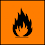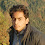## Saturday, November 12, 2011

### Rotate an Object in OpenGL(GLUT) sample example with source

In GLUT we rotate the object by glRotate3f(angle_rotation,GLfloat x, GLfloat y ,GLfloat z) function angle_rotation parameter is value of angle to rotate and x,y,z co-ordinate are axis of rotation.But it does not rotate object continuously for that we have to change angle of rotation by small amount of increment. We do this by using timer thread with the help of glutTimerFunc function.Here is the sample source code copy and compile it.

`#include<windows.h>`
`#include <GL/glut.h>`
`#include<iostream>`
`#include <stdlib.h>`
` `
`using namespace std;`
` `
`void handleKeypress(unsigned char key, int x, int y)`
`{`
`switch(key)`
`{`
`case 27:`
`exit(0);`
`}`
`}`
`void initRendering()`
`{`
`glEnable(GL_DEPTH_TEST);`
`}`
` `
`void handleResize(int w,int h)`
`{`
`glViewport(0,0,w,h);`
`glMatrixMode(GL_PROJECTION);`
`glLoadIdentity();`
`gluPerspective(45.0,(double)w/(double)h,1.0,200.0);`
`}`
` `
`float _angle=0.0;`
`float _cameraangle=30.0;`
` `
`void drawScene()`
`{`
` `
` `
` `
`glClear(GL_COLOR_BUFFER_BIT|GL_DEPTH_BUFFER_BIT);`
`glMatrixMode(GL_MODELVIEW);`
`glLoadIdentity();`
`glRotatef(_cameraangle,0.0f,1.0f,0.0f); //rotate object by 30 degree with respect to y-axis`
`glTranslatef(0.0f, 0.0f, -10.0f);`
` `
`glPushMatrix();`
`glTranslatef(5.0f, -1.0f, 0.0f);`
`glScalef(2.0f,2.0f,2.0f);`
`glRotatef(_angle,1.0f,3.0f,2.0f); //rotating object continuously by 2 degree`
`glBegin(GL_QUADS);`
` `
`glVertex3f(-0.7f,0.0f,0.0);`
`glVertex3f(0.7f,0.0f,0.0);`
`glVertex3f(0.5f,2.0f,0.0);`
`glVertex3f(-0.5f,2.0f,0.0);`
` `
`glEnd();`
` `
`glPopMatrix();`
`glutSwapBuffers();`
` `
` `
`}`
`void update(int value)`
`{`
`_angle+=2.0f;`
`if(_angle>360.f)`
`{`
`_angle-=360;`
`}`
`glutPostRedisplay();`
`glutTimerFunc(25,update,0);`
`}`
` `
`int main(int argc,char**argv)`
`{`
`glutInit(&argc,argv);`
`glutInitDisplayMode(GLUT_DOUBLE|GLUT_RGB|GLUT_DEPTH);`
`glutInitWindowSize(600,600);`
` `
` `
`glutCreateWindow("rotate");`
`initRendering();`
` `
` `
` `
`glutDisplayFunc(drawScene);`
`glutKeyboardFunc(handleKeypress);`
`glutReshapeFunc(handleResize);`
` `
`glutTimerFunc(25,update,0);`
`//this call the function update in every 25 millsecond`
`//so value of angle of rotation change and object rotates continuously`
`glutMainLoop();`
` `
` `
`return 0;`
`}`

1.This comment has been removed by the author.

2.Greate!

One little thing. Without the two lines

#include < iostream >

and

using namespace std;

this is valid C ;)

1.you are right

3.hello...i wated a cpp code using opengl for the animation of globe and cube with text rotation.Can any help mw out with this...thank u..regards:)

4.can u plz give me a code of alphabets animation..like if A appears on screen an apple also appears and a background sound of pronounciation of A?